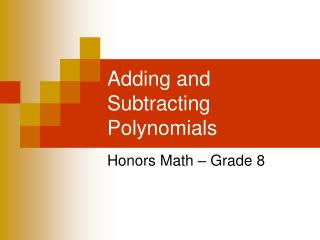DownloadDownload PresentationTélécharger la présentation- - - - - - - - - - - - - - - - - - - - - - - - - - - E N D - - - - - - - - - - - - - - - - - - - - - - - - - - -
##### Presentation Transcript

2. Study Tip When adding polynomials, remember to use the integer rules! Add Polynomials Find Method 1 Method 2 (3x2 – 4x + 8) + (2x – 7x2 – 5) Write in column form. Align like terms. Group like terms Add Add like terms

3. Study Tip When adding polynomials, remember to use the integer rules! Add Polynomials Find Method 1 Method 2 (7y2 + 2y -3) + (2 – 4y + 5y2) Write in column form. Align like terms. Group like terms Add Add like terms

4. To subtract polynomials: Add the opposite (AKA the additive inverse) Subtract Polynomials Find Method 1 (3n2 + 13n3 + 5n) - (7n + 4n3) Subtract by adding the additive inverse (3n2 + 13n3 + 5n) + (-7n - 4n3) Polynomial Additive Inverse Group like terms Combine like terms

5. To subtract polynomials: Study Tip Add the opposite (AKA the additive inverse) Don’t forget the integer rules! Subtract Polynomials Find Method 2 Align like terms in columns and subtract by adding the additive inverse. Add the opposite

6. To subtract polynomials: Study Tip Add the opposite (AKA the additive inverse) Don’t forget the integer rules! Subtract Polynomials Find Method 2 Align like terms in columns and subtract by adding the additive inverse. Add the opposite

7. Real World Example – EDUCATION The total number of public school teachers T consists of two groups, elementary E and secondary S. From 1992 through 2003, the number (in thousands) of secondary teachers and total teachers could be modeled by the following equations, where n is the number of years since 1992. Find an equation that models the number of elementary teacher E for this time period. What is known? Add the opposite Write an equation 29n + 1452 An equation is E = 29n + 1452 Use the equation to predict the number of elementary teacher is 2015. How many years from 1992 is 2015? 2015 – 1992 = 23 years Plug in n = 23 n represents the number of years since 1992. The number of teachers would be 2,119,000

8. GEOMETRY Given the perimeter of the triangle, find the third side. Recall that perimeter is the total distance around a polygon. To find the perimeter of a figure, add the lengths of all the sides. Add the two sides that are known. This represents the sum of two of the sides. How do you find the third side? Subtract The length of the third side is (4x + 2y) units.